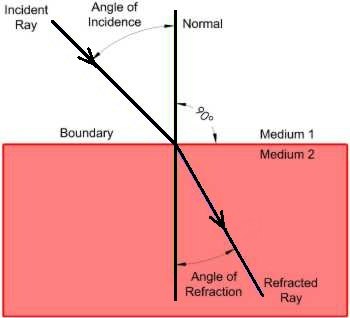# Light - Physics Help | Optics | Physics Assignment Help

## What is Light?

Light is that form of energy or portion of electromagnetic radiation which makes object visible to our eye. The branch of physics which deals with the nature of light, its sources, properties, effects and vision is called optics.

In other words we define optics - the branch of physics that studies the physical properties of light. Today optics is an important research area in modern physics. The study of optics is divided into two main fields namely geometrical optics and physical optics.

Geometrical optics treats propagation of light in terms of rays and is valid only if wavelength of light is much lesser than the size of obstacles. It concerns with the image formation and deals with the study of simple facts such as rectilinear propagation, laws of reflection and refraction by geometrical methods.

Physical optics is further divided into two parts namely wave optics and quantum optics. Wave optics concerns with explanation of observed phenomena such as diffraction, interference, and polarization by treating light as a wave. Quantum optics treats light as particles called "photon". In order to explain several phenomena associated with emission and absorption of light such as Photoelectric effect, Compton effect, Raman effect, and LASER etc.

Planck Relation (E = hv) and Einstein theory of relativity (E = mc2) [Albert Einstein developed a theory about the relationship of mass and energy.] developed "quantum theory of light".

Electromagnetic radiation has a dual nature as both particles and waves. One way to look at it is as changing electric and magnetic fields which propagate through space, forming an electromagnetic wave. This wave has amplitude, which is the brightness of the light, wavelength, which is the color of the light, and an angle at which it is vibrating, called polarization.

### Refraction of Light:

Light bends when it moves at an angle from one transparent substance, such as air, to another substance, such as water. This bending of light is called refraction. We call the substances that light can move through mediums. Water, glass, and air are mediums. Light refracts at different angles depending on the density of the medium. The angle between the light ray and the normal as it leaves a medium is called the angle of incidence. The angle between the light ray and the normal as it enters a medium is called the angle of refraction.The index of refraction, n, is a ratio that compares the speed of light in a vacuum to the speed of light in another medium. The speed of light in all other media is less than in a vacuum. Therefore, n is always larger than one.

n = c/v where c = speed of light in a vacuum = 3 X 108 m/s

In optics, refraction occurs when waves travel from a medium with a given refractive index to a medium with another at an angle. At the boundary between the media, the wave's phase velocity is altered, usually causing a change in direction. Its wavelength increases or decreases but its frequency remains constant.

Refraction is the bending of light that takes place at a boundary between two mediums having different indices of refraction. The boundary is where one medium meets another medium. Refraction is due to a change in the speed of light as it passes from one medium to another. Given figure illustrates this concept.

The angles of incidence and refraction are measured relative to a line perpendicular to the boundary between the mediums called the normal. The mediums that the light passes from and to are transparent. The light will bend based on a relationship called snell's Law.

Figure: Refraction of Light

### Snell's Law:

In 1621, a Dutch physicist named Willebrord Snell (1591-1626), derived the relationship between the different angles of light as it passes from one transparent medium to another. When light passes from one transparent medium to another, it bends according to snell's law which states:

n1sinø1 = n2sinø2

where:
n1 is the refractive index of the medium the light is leaving.
ø1 is the incident angle between the light ray and the normal to the medium to medium interface.
n2 is the refractive index of the medium the light is entering.
ø2 is the refractive angle between the light ray and the normal to the medium interface.

The incident and refracted rays are on opposite sides of the normal at the point of incidence. The incident ray, the normal, and the refracted ray are coplanar. As the light slows down it will bend toward the normal line and as it speeds up it will bend away.

The absolute index of refraction for a given medium is defined as: 直 = c/v where c is the speed of light in a vacuum and v is the speed of light in the medium. The refractive index of a vacuum is 1.### Physics Assignment Help Services (Live (24/7) Tech. Support)

Assignmenthelp.net is one the leading online physics tutoring service provider in e-education. You can find answers to all of your doubts in Physics (light - questions and answers, electromagnetic radiation, optics, refraction of light, and snell's law ). Online physics tutors are ready to help you based on your physics queries and problems. Here, students can find best solutions of their physics problems and related subjects.

To submit Physics (light, optics, refraction of light, and snell's law) assignments/problems click here